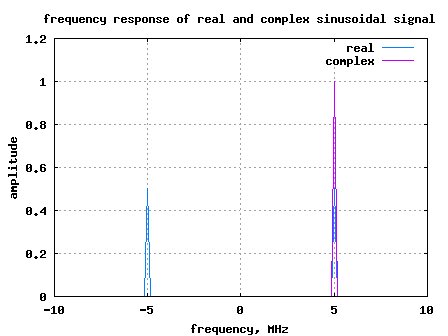- DSP log - http://www.dsplog.com -

Negative Frequency

Posted By Krishna Sankar On August 8, 2008 @ 6:05 am In DSP | 25 Comments

Last week, I received an email from Mr. Kishore. He was wondering about the physical significance of negative frequency. Does negative frequency really exist?

Though I have seen conflicting views on the net (thread in complextoreal.com , thread in comp.dsp ), my perspective is that negative frequency exist. The concept of negative frequency helps me a lot to understand single sideband modulation (SSB), OFDM systems, I Q modulators etc (to name a few).

## Simple explanation for negative frequency

The wiki entry on negative frequency  provides a simple explanation using as an example.

We know that . This means the sign of cannot be un-ambiguously found out from observing alone. This implies that it is reasonable to think that has frequency components at both and .

Similarly, this ambiguity exists for too.

## Negative frequency using Taylor’s series expansion

Thanks to the nice paper by Mr. Richard Lyons, Quadrature Signals: Complex but not Complicated, Richard Lyons .

Let us first define the magic number and so on.

The Taylor series expansion of , and are as follows.

Let us now define the Taylor series expansion of .

.

Similarly,

.

Combining the above two equations, one may write

.

If we apply , then we get,

and

.

This forms the proof that a real sinusoidal having frequency is comprised of a complex sinusoidal having a positive frequency at and a negative frequency at .

## Simple Matlab example for Negative frequencyFigure: Spectrum plot showing positive and negative frequency

As discussed above, the real sinusoidal has frequency components at +5MHz and -5MHz where as the complex sinusoidal has frequency component only at +5MHz.

## Negative frequency in OFDM

Some of you might be familiar with the IEEE 802.11a specification  where subcarriers from [-26 to -1 and [+1 to 26] are used. The subcarriers -26 to -1 corresponds to usage of negative frequency and lets try to understand it.

The equation for an OFDM transmission is,

,

where

(a) correspond to the frequency of the sinusoidal and

(b) is a rectangular window over

(c) is the symbol period.

(d) each information signal is modulated on to a complex sinusoidal having frequency of .

(e) Sum of all such modulated sinusoidals are added and the resultant signal is sent out as .

In the IEEE 802.11a specification , symbol duration is 3.2, sampling frequency is 20MHz and .

The frequencies used for modulating the ‘s are , , , and so on till . Expressing in Hz, this corresponds to frequencies from 0Hz, 312.5kHz, 625kHz, 937.5kHz, 1.25MHz,…, 10MHz, 10.3125MHz,… till 19.6875MHz.

From our understanding of sampling theory, we know that with a sampling frequency of , we can only see frequencies from to .

Note: The frequency is called the Nyquist frequency. 

So in our 802.11a example, what will happen to frequencies which are modulated on subcarriers lying from 10MHz till 19.6875MHz?

Quick answer: They get folded!The frequencies from 10MHz till 19.6875MHz gets folded and seems as if they are lying from -10MHz to -312.5kHz.Figure: Spectrum folding to negative frequency in IEEE802.11a specification

Further, folding of the spectrum to the negative frequency region does not cause any problems. Reason: ‘s which where modulated on complex sinusoidals having frequencies from 0 till 10MHz did not have any negative frequency component.

Note:

In general, one can say if a sinusoidal is of frequency is sampled with a frequency and if , the frequency gets folded to a frequency within , where is an integer.

The concept of folding is well explained in Chapter 1.4.1 of [DSP: PROAKIS]. A simple example of folding which we may see in our day to day life is with a ceiling fan. One may see that the blades of the fan are rotating at a slower speed in a direction opposite to the actual rotation of the fan blades. Needless to say that the sampling frequency of our eye’s are not good enough.Happy learning.

## Reference

[DSP: PROAKIS]: Digital Signal Processing, John G. ProakisURL to article: http://www.dsplog.com/2008/08/08/negative-frequency/

URLs in this post:

 wiki entry on negative frequency: http://en.wikipedia.org/wiki/Negative_frequency